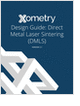Design HomeSensorsSensor HomeInstruments/DevicesMethods/PrinciplesDisplacementStress & StrainPressureFluid FlowFlowmeterIntroductionCategorizationSelectionCoriolisDifferential PressureMagneticPositive DisplacementTargetThermalTurbineUltrasonicTransit TimeDopplerVariable AreaVortexTemperatureResourcesBibliography
Metal 3D Printing Design Guide

Direct Metal Laser Sintering (DMLS) 3D printing for parts with reduced cost and little waste.

3D Scanners

A white paper to assist in the evaluation of 3D scanning hardware solutions.

Salary Expectation

8 things to know about the interview question "What's your salary expectation"?

Injection Molding Design Guide

Guide for high quality and cost-effective plastic injection molding.

more free publicationsOverviewA Variable area flowmeter's cross section area available to the flow varies with the flow rate. Under a (nearly) constant pressure drop, the higher the volume flow rate, the higher the flow path area. Variable area flowmeters can further be categorized into three types Rotameter: The most widely used variable area flowmeter.Movable Vane Meter:Weir, Flume:Variable area flowmeters are robust, low cost, simple, however less accurate, compared to other more complicated flowmeters.
 Further InformationRotameter is the most widely used variable area flowmeter. Its principle of operation is discussed below. A movable vane meter has similar principle of operation, except the "moving piston (float)" of rotameter is now a swing open valve and the weight of the float is replaced by the spring force applied on the valve. Rotameter: A rotameter is mounted vertically with the narrow end at the bottom and the tube tappers into a wider top. The flow comes from the bottom and pushes the float inside the rotameter up to a point that the weight of the float is in balance with the force exerted by the flow. The annular area between the float and the tube wall is then related to the volume flow rate. As long as the fluid speed is substantially subsonic (V < mach 0.3), the incompressible Bernoulli's equation applies.where g is the gravity acceleration constant (9.81 m/s2 or 32.2 ft/s2), V is the velocity of the fluid, and z is the height above an arbitrary datum. C remains constant along any streamline in the flow, but varies from streamline to streamline. If the flow is irrotational, then C has the same value for all streamlines. Applying this equation to a streamline traveling up the axis of the vertical tube gives,where subscript a represents the position right below the float, b is the balanced point of the float, usually the top of the float, V is the flow velocity, p is pressure, andis the density. A shorter form of the above equationi iswhere hf is the hight of the float or the distance from the bottom to the indicator of the float that depends on the float design. From continuity, the volume flow rate at a is the same as the volume flow rate at b, i.e.,, which impliesPlease note thatis the annular area between the float and the tube wall, not the whole cross section area at b. Hence, the velocity Vb can be substituted out of the Bernoulli's equation to give,The pressure drop is mostly resulting from the weight of the floatwhere the subscript f represents the float, Vf is the volume, Af is the cross section area, andf is the density of the float. Solving for the volumetric flow rate Q, we haveIdeal, inviscid fluids would obey the above equation. The small amount of energy converted into heat within viscous boundary layers tends to somewhat lower the actual velocity of real fluids. A discharge coefficient C is typically introduced to account for the viscosity of fluids,C is found to depend on the Reynolds Number of the flow. For a given design, the cross section areas Aa(z) and Ab(z) of the rotameter are functions of the hight z, and the geometry (hf, Af, Vf) and the density (f) of the float are also known. If the density of the fluidis measured and the readout of the position (z) of the float in the rotameter is available, the volume flow rate Q can be calculated from this formula:The mass flow rate can be easily found by multiplying Q with the fluid density,Common SpecificationsCommon specifications for commercially available variable area flowmeters are listed below:

Fluid Phase: Please refer to flowmeter selection for specific sub-categories

 Score Phase ConditionGas CleanLiquid CleanOpen ChannelGas DirtyLiquid CorrosiveDirtySteam Saturated: Recommended: Limited applicability
Line Size: Mostly used in lines size 100 mm (4 inch) and below, but may go up to 2000 mm (80 inch) in line size or even for open channels.
Turndown Ratio: 10 ~ 100 : 1
Pros and Cons• Pros: - Very low initial set up cost - Simple, robust - Low, nearly constant, pressure drop • Cons: - Moderate accuracy at best - Not suitable for low flow rate - Some variable area flowmeters can not be used in non/low gravity environments - Rotameters must be mounted vertically
GlossaryMetal 3D Printing Design Guide

Direct Metal Laser Sintering (DMLS) 3D printing for parts with reduced cost and little waste.3D Scanners

A white paper to assist in the evaluation of 3D scanning hardware solutions.Salary Expectation

8 things to know about the interview question "What's your salary expectation"?Injection Molding Design Guide

Guide for high quality and cost-effective plastic injection molding.